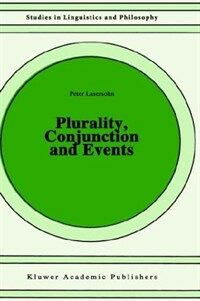> 상세정보

# 상세정보## Plurality, conjunction, and events (5회 대출)

자료유형
단행본
개인저자
Lasersohn, Peter.
서명 / 저자사항
Plurality, conjunction, and events / by Peter Lasersohn.
발행사항
Dordrecht ;   Boston :   Kluwer Academic Publishers ,   c1995.
형태사항
xi, 304 p. ; 23 cm.
총서사항
Studies in linguistics and philosophy ; v. 55
ISBN
0792332385 (hbk.) 9780792332381 (hbk.)
서지주기
Includes bibliographical references (p. 290-304).
일반주제명
Grammar, Comparative and general -- Number. Grammar, Comparative and general -- Conjunctions. Semantics.
 000 00981camuu2200289 a 4500 001 000045456330 005 20080723144004 008 941006s1995 ne b 000 0 eng 010 ▼a 94038629 020 ▼a 0792332385 (hbk.) 020 ▼a 9780792332381 (hbk.) 035 ▼a (KERIS)REF000014119828 040 ▼a DLC ▼c DLC ▼d DLC ▼d 211009 050 0 0 ▼a P240.8 ▼b .L37 1995 082 0 0 ▼a 415 ▼2 22 090 ▼a 415 ▼b L343p 100 1 ▼a Lasersohn, Peter. 245 1 0 ▼a Plurality, conjunction, and events / ▼c by Peter Lasersohn. 260 ▼a Dordrecht ; ▼a Boston : ▼b Kluwer Academic Publishers , ▼c c1995. 300 ▼a xi, 304 p. ; ▼c 23 cm. 440 0 ▼a Studies in linguistics and philosophy ; ▼v v. 55 504 ▼a Includes bibliographical references (p. 290-304). 650 0 ▼a Grammar, Comparative and general ▼x Number. 650 0 ▼a Grammar, Comparative and general ▼x Conjunctions. 650 0 ▼a Semantics. 945 ▼a KINS

### 소장정보

No. 소장처 청구기호 등록번호 도서상태 반납예정일 예약 서비스
No. 1 소장처 청구기호 415 L343p 등록번호 111482677 도서상태 대출가능 반납예정일 예약 서비스

### 컨텐츠정보

#### 목차

```
CONTENTS
Preface = ⅸ
Part One. Collective and Distributive Readings: History and Range of Possible Analyses = 1
Introduction to Part One: An Apparent Difference Between Two Types of Conjunction = 2
Chapter 1: Logical Form = 4
1.1. Two Notions of Logical Form = 4
1.2. Two Notions of Compositionality = 5
1.3. Compositionality and Logical Form = 7
1.4. Syntactic Motivation for LF = 10
Notes = 11
Chapter 2: The NP/S Analysis = 12
2.1. Historical Notes on the NP/S Analysis = 12
2.2. Conjunction Reduction = 17
2.3. The NP/S Analysis: Formal Presentation = 20
Notes = 24
Chapter 3: The Relational/S Analysis = 26
Notes = 32
Chapter 4: The S/S Analysis = 33
4.1. Historical Notes on the S/S Analysis = 33
4.2. "Official Version" and Critique of the  S/S Analysis = 50
Notes = 51
Chapter 5: Two Types of NP/NP Analysis = 55
5.1. Primitive Phrasal and Generalized  Conjunction = 55
5.2. Conjoined Noun Phrases and Plural Noun Phrases = 64
Notes = 68
Chapter 6: Interlude On Collective Action and Colors = 69
Notes = 80
Chapter 7: Locating the Ambiguity = 81
7.1. The Group-Level Analysis of Distributivity = 83
7.2. Testing for Ambiguity = 89
7.3. First Problem for Noun Phrase Ambiguity Accounts: Failure of the Zeugma Test = 96
7.4. Second Problem for Noun Phrase Ambiguity Accounts: Partial Distributivity and Participatory Distributivity = 104
7.5. Third Problem for Noun Phrase Ambiguity Accounts: Verb Agreement = 109
7.6. Problems with Mode-of-Composition Analyses = 114
7.7. Problems with Scope Ambiguity Analyses = 116
7.8. Conclusion = 124
Notes = 124
Chapter 8: How Many Readings? = 128
8.1. Just One = 128
8.2. Arbitrarily Many = 132
8.3. Two = 141
Notes = 144
Chapter 9: On The Structure of Groups = 145
9.1. Sets and Groups, Union and Pairing = 116
9.2. Conjunctions of Singulars = 147
9.3. The Sets Theory and the Union Theory  Point to Different Ontologies = 148
9.4. The Argument from 'Both' = 150
9.5. The Argument from Reciprocals = 151
9.6. The Argument from Distributives  = 153
9.7. The Argument from 'Same' and 'Different' = 155
9.8. First Response to the Arguments: Pure and Impure Atoms = 156
9.9. Second Response to the Arguments: Covers = 159
9.10. Conclusion = 167
Notes = 167
Chapter 10: The Event-Free Fragment = 169
10.1. Basic Claims  = 169
10.2. Syntax = 170
10.3. Semantics = 172
Notes = 177
Part Two. Events in the Semantics of Plurality and Conjunction = 179
Introduction to Part Two: Extending the Coverage = 180
Chapter 11: Earlier Work on Collectivizing Adverbials = 182
11.1. A Problem in Distinguishing Collective and Distributive Predicates = 182
11.2. An Analysis Based on the Scope of an Event Quantifier = 185
11.3. An Analysis Based on Event Mereology = 189
11.4. Spatial and Temporal Readings = 200
11.5. An Analysis Based on Predicate Negation = 205
Notes = 214
12.1. Getting Clear about the Problem = 218
12.2. Analyzing 'Together' in Terms of Overlap = 222
12.3. Persistence and Quantification = 226
Notes = 237
Chapter 13: Pluractional Markers = 238
13.1. Introduction = 238
13.2. More than Just Groups of Events = 241
13.3. Parameters of Pluractionality = 242
13.4. Skeleton of an Analysis = 251
13.5. Pluractionality in Klamath = 257
Notes = 264
Chapter 14: Unifying the Semantics of Conjunction = 267
14.1. Groups of Events = 267
14.2. A Problem with Temporal Alternation = 268
14.3. Alternation and Groups of Events = 272
14.4. Conjunction and Groups of Events = 277
14.5. Color and Material Conjunction = 281
Notes = 286
Conclusion = 287
References = 290

```

### 관련분야 신착자료

#### Grammaticalization / 1st ed

Narrog, Heiko (2021)